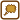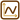## Steel ball

We will release a steel ball from rest down a smooth angled plane upon which it moves with acceleration of 0,5 m·s−2. Then it moves to a level plane. In total, it travels 20 meters in 12 seconds. For how long did it move on the angled plane?

Neglect friction of the environment and the plane.

• #### List of known information

 a = 0,5 m·s−2 ball’s acceleration s =  20 m total travelled distance t = 12 s total time of balls movement t1 = ? (s) time of ball’s movement on the angled plane
• #### Hint 1: Distance and speed of ball on angled plane

Draw a picture and mark all needed quantities.

What distance will the ball travel on the angled plane and what speed will it reach?

• #### Hint 2: Ball’s movement on level plane

What is the ball’s movement on the level plane?

What is its speed during this movement?

What distance does it travel?

• #### Hint 3: Total distance of the ball

Express the total distance the ball has travelled.

Solve the quadratic equation that you get for time t1 and figure out which root of the equation fits the task’s conditions.

• #### Overall Solution

Picture and marking of quantities:The ball moves on the angled plane with a uniformly accelerated straightforward motion. Then, on the level plane, it moves with a uniform straightforward motion. On the angled plane, the ball moves for t1 with a set acceleration a. In this time it travels the distance of:

$s_1\,=\,\frac{1}{2}at_1^{2}\,.$

And will reach the speed:

$v\,=\,at_1\,.$

It will move on the level plane with this speed for time of t - t1 and will travel the distance:

$s_2\,=\,v\left(t-t_1\right)\,=\,at_1\left(t-t_1\right)\,.$

Total distance will be:

$s\,=\,\frac{1}{2}\,at_1^{2}\,+\,at_1\left(t-t_1\right)\,.$

We multiply the brackets:

$s\,=\,-\frac{1}{2}\,at_1^{2}\,+\,at_1t\,.$

$t_1^{2}\,-\,2tt_1\,+\,\frac{2s}{a}\,=\,0\,.$

By solving the quadratic equation, we get two roots (marked as t1, t2); only one fits:

$t_1\,=\,t\,-\,\sqrt{t^{2}\,-\,\frac{2s}{a}}\,.$

Because:

$t_1\,<\,t\,.$

Numerically:

$t_1\,=\,\left(12\,-\,\sqrt{(12)^{2}\,-\,\frac{2{\cdot} 20}{0{,}5}}\right)\,\mathrm{s}\,=\,4\,\mathrm{s}\,.$
$t_1\,=\,t\,-\,\sqrt{t^{2}\,-\,\frac{2s}{a}}\,=\, 4\,\mathrm{s}\,.$